# Multisim and Ultiboard

cancel
Showing results for
Did you mean:

## Logic analyzer and JK asynchronous flip-flop operation

I have such a simple diagram of a JK asynchronous flip-flop along with a signal plot in a logic analyzer. I have two questions:

• How do I modify the run speed and range on the logic analyzer? I mean this chart is moving very fast, how do I slow it down and increase the range?
• The second question, according to the theory, this flip-flop should count the signal up and it counts the signal down. Why is this the case seems to be correct to me.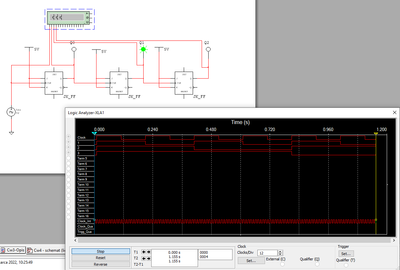Message 1 of 14
(586 Views)

## Re: Logic analyzer and JK asynchronous flip-flop operation

ms- файл в студию, плиз!

Message 2 of 14
(574 Views)

## Re: Logic analyzer and JK asynchronous flip-flop operation

The file is in the attachment.

Message 3 of 14
(563 Views)

## Re: Logic analyzer and JK asynchronous flip-flop operation

шаг моделирования надо увеличить, чтобы повысить быстродействие анимации и быстроту счёта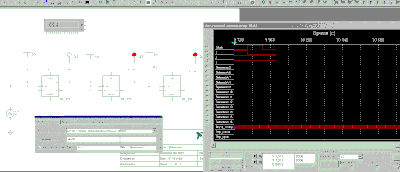Message 4 of 14
(560 Views)

## Re: Logic analyzer and JK asynchronous flip-flop operationнадеюсь, чем-то помог, если я вас правильно понял...

Message 5 of 14
(555 Views)

## Re: Logic analyzer and JK asynchronous flip-flop operationUnfortunately for me it's still the same. I change the options that you are talking about, after all, after 1.280 sec, the plot on graph starts from the beginning. I can change "clocks / div", also change in "Interactive simulation" -> "End time (TSTOP)" to larger values (eg 1e + 60) and still the same.
Maybe record a video from the beginning so I can trace exactly what you change, it seems to me that I miss something or I do not fully understand something.
I don't speak Russian at all, I don't know why you write to me in this language if you understand English...

And I still don't understand one thing: this circuit is built exactly like the book example of an asynchronous counter on JK flip-flops, which should count the signal up and in my case it counts down. Why is this happening?

Here is the comparison between "book example" and mine.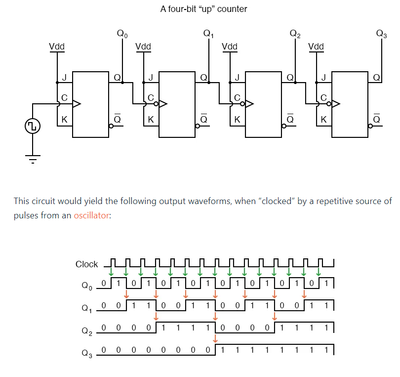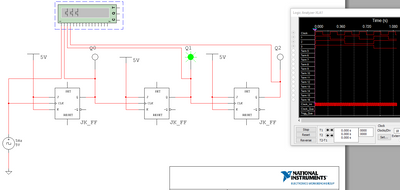Message 6 of 14
(473 Views)

## Re: Logic analyzer and JK asynchronous flip-flop operation

Cool, I've found the answer to the first part of my question, is here by increasing number of points.However I still don't know why this flip-flop counts time down.

In this "Clock voltage" element, it takes a positive value at the very beginning of the duty cycle, maybe it should be negative first, then positive? I just don't know how to to change it.

Message 7 of 14
(461 Views)

## Re: Logic analyzer and JK asynchronous flip-flop operation

Я рад за вас, что вы смогли продвинуться в своих попытках, по крайней мере в какой-то степени. Мой английский застопорился на уровне 5-го класса (1969). Типа: "Привет, Георг! Я рад тебя видеть! Мне нужно многое тебе рассказать. Мой друг Пит, который живет в Лондоне...и так далее.
Когда что-то не получается или у нас не хватает мозгов для какого-то решения, они говорят так:
"Ты не можешь понять это без бутылки".🙂

Message 8 of 14
(456 Views)

## Re: Logic analyzer and JK asynchronous flip-flop operation

Hi devoreaux1,

The counter depicted in your "book example" is called a RIPPLE UP COUNTER. In this kind of counter the FLIP-FLOP for Least Significant Bit (LSB) is toggled by Clock source, succeeding FLIP-FLOPs are clocked from the output of preceding stage.

In the "book example" the clock (C) input of the first flip-flop is connected to the Clock source. Here, there is no discrepancy between the "book example" and your Multisim circuit. Note, however, that in the succeeding stages the J-K flip-flops are NEGATIVE EDGE-TRIGGERED which are distinguished by BUBBLE (circle) in clock input (C) terminal. To make the circuit work as up counter Q0 is used to clock the flip-flop for bit 1, Q1 is used to clock the flip-flop for bit 2, and Q2 is used to clock the flip-flop for bit 3.

To make your circuit work, you can use one of the corrections below:

• Use NEGATIVE-EDGE TRIGGERRED J-K flip-flops except for the first stage (carefully check the image from your book example, the first stage is POSITIVE EDGE-TRIGGERRED while the rest are NEGATIVE EDGE-TRIGGERRED). If you use negative edge-triggered flip-flop for the first stage, the circuit will work but the counter will advance on the falling edge of clock source (Clock).
• Keep the POSITIVE EDGE-TRIGGERRED J-K flip-flops that you used in your circuit but wire the clock input of the SECOND and succeeding stages from NOT Q (~Q) output of the preceding stage. This means, use NOT Q0 (~Q0) to clock the flip-flop for bit 1, use NOT Q1 (~Q1) to clock the flip-flop for bit 2, and use NOT Q2 (~Q2) to clock the flip-flop for bit 3. The output count should remain taken from Q output terminals (Q3, Q2, Q1, and Q0).

I am only a Free Subscription user of Multisim Live, I don't have access to sequential devices (latches and flip-flops) so I can't construct the correct circuit for you.

The computer that I'm using does not have an image editor so I can't encircle the parts that I'm referring to in the images that you attached.

Best regards,
G. Goodwin

Message 9 of 14
(423 Views)

## Re: Logic analyzer and JK asynchronous flip-flop operation

Thank you for your in-depth problem analysis. In fact, in this "book" example from the internet, I didn't notice that the clock inputs are inverted. It follows that when using individual flip-flops, you have to look at their principle of operation.
Nevertheless, I decided to look at a more professional source, ie the book Electronic Circuits: Handbook for Design and Application by Tietze, Ulrich; Schenk, Christoph.
And here is an example:

There are no negated clock inputs here. The output is directly from Q and the description is as follows:A straight binary asynchronous (ripple) counter can be implemented by arranging toggle flip-flops in a chain, as shown in Fig. 9.29, and by connecting each clock input C to the output Q of the previous flip-flops. If the circuit is to be an up-counter, the flip-flops must change their output states when their clock inputs C change from 1 to 0. Edge-triggered flipflops are therefore required; for example, JK master–slave flip-flops where J = K = 1. The counter may be extended to any size. Using this principle, one can count up to 1,023 with only ten flip-flops [...]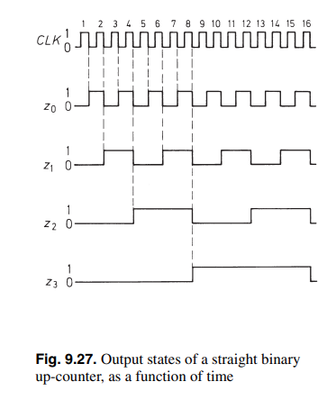So from what I can see this flip-flop works the opposite way to my example. For me, there was a change of state on the flip-flop when the clock changed from zero to one.

Message 10 of 14
(387 Views)